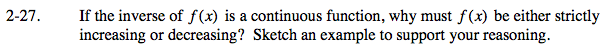### Home > CALC > Chapter Ch2 > Lesson 2.1.2 > Problem2-27

2-27.

If the inverse of f(x) is a continuous function, why must f(x) be either strictly increasing or decreasing? Sketch an example to support your reasoning. Homework Help ✎Strictly increasing means, as x increases, y-values are always going up.
Strictly decreasing means, as x increases, y-values are always going down.

If a function is NOT strictly increasing or decreasing, then either
I. it oscillates between increasing and decreasing
II. it is horizontal always or sometimes

As you sketch, try to find a counter-example to this statement. In other words, try to sketch a function with a continuous inverse function that is NOT strictly increasing or strictly decreasing.

Note that f(x) and its inverse must both be continuous AND both be functions.